2022: SklogWiki celebrates 15 years on-line

# Grand canonical ensemble

The grand-canonical ensemble is for "open" systems, where the number of particles,$N$, can change. It can be viewed as an ensemble of canonical ensembles; there being a canonical ensemble for each value of$N$, and the (weighted) sum over$N$ of these canonical ensembles constitutes the grand canonical ensemble. The weighting factor is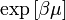$\exp \left[ \beta \mu \right]$ and is known as the fugacity. The grand-canonical ensemble is particularly well suited to simulation studies of adsorption.

## Ensemble variables

• chemical potential,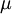$\left. \mu \right.$
• volume,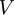$\left. V \right.$
• temperature,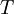$\left. T \right.$

## Grand canonical partition function

The grand canonical partition function for a one-component system in a three-dimensional space is given by: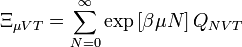$\Xi_{\mu VT} = \sum_{N=0}^{\infty} \exp \left[ \beta \mu N \right] Q_{NVT}$

where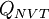$Q_{NVT}$ represents the canonical ensemble partition function. For example, for a classical system one has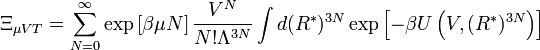$\Xi_{\mu VT} = \sum_{N=0}^{\infty} \exp \left[ \beta \mu N \right] \frac{ V^N}{N! \Lambda^{3N} } \int d (R^*)^{3N} \exp \left[ - \beta U \left( V, (R^*)^{3N} \right) \right]$

where:

•$N$ is the number of particles
•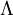$\left. \Lambda \right.$ is the de Broglie thermal wavelength (which depends on the temperature)
•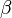$\beta$ is the inverse temperature
•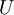$U$ is the potential energy, which depends on the coordinates of the particles (and on the interaction model)
•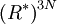$\left( R^*\right)^{3N}$ represent the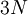$3N$ position coordinates of the particles (reduced with the system size): i.e.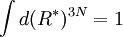$\int d (R^*)^{3N} = 1$

## Helmholtz energy and partition function

The corresponding thermodynamic potential, the grand potential,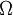$\Omega$, for the aforementioned grand canonical partition function is: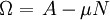$\Omega = \left. A - \mu N \right.$,

where A is the Helmholtz energy function. Using the relation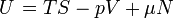$\left.U\right.=TS -pV + \mu N$

one arrives at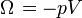$\left. \Omega \right.= -pV$

i.e.: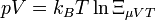$\left. p V = k_B T \ln \Xi_{\mu V T } \right.$